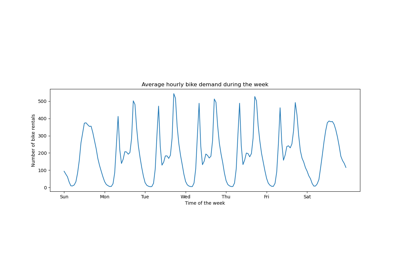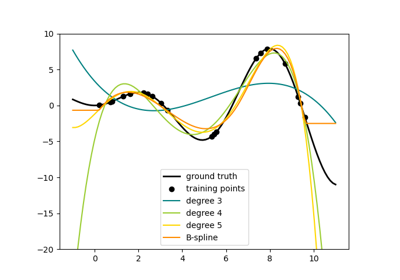# sklearn.preprocessing.SplineTransformer¶

class sklearn.preprocessing.SplineTransformer(n_knots=5, degree=3, *, knots='uniform', extrapolation='constant', include_bias=True, order='C')[source]

Generate univariate B-spline bases for features.

Generate a new feature matrix consisting of n_splines=n_knots + degree - 1 (n_knots - 1 for extrapolation="periodic") spline basis functions (B-splines) of polynomial order=degree for each feature.

Read more in the User Guide.

New in version 1.0.

Parameters:
n_knotsint, default=5

Number of knots of the splines if knots equals one of {‘uniform’, ‘quantile’}. Must be larger or equal 2. Ignored if knots is array-like.

degreeint, default=3

The polynomial degree of the spline basis. Must be a non-negative integer.

knots{‘uniform’, ‘quantile’} or array-like of shape (n_knots, n_features), default=’uniform’

Set knot positions such that first knot <= features <= last knot.

• If ‘uniform’, n_knots number of knots are distributed uniformly from min to max values of the features.

• If ‘quantile’, they are distributed uniformly along the quantiles of the features.

• If an array-like is given, it directly specifies the sorted knot positions including the boundary knots. Note that, internally, degree number of knots are added before the first knot, the same after the last knot.

extrapolation{‘error’, ‘constant’, ‘linear’, ‘continue’, ‘periodic’}, default=’constant’

If ‘error’, values outside the min and max values of the training features raises a ValueError. If ‘constant’, the value of the splines at minimum and maximum value of the features is used as constant extrapolation. If ‘linear’, a linear extrapolation is used. If ‘continue’, the splines are extrapolated as is, i.e. option extrapolate=True in scipy.interpolate.BSpline. If ‘periodic’, periodic splines with a periodicity equal to the distance between the first and last knot are used. Periodic splines enforce equal function values and derivatives at the first and last knot. For example, this makes it possible to avoid introducing an arbitrary jump between Dec 31st and Jan 1st in spline features derived from a naturally periodic “day-of-year” input feature. In this case it is recommended to manually set the knot values to control the period.

include_biasbool, default=True

If True (default), then the last spline element inside the data range of a feature is dropped. As B-splines sum to one over the spline basis functions for each data point, they implicitly include a bias term, i.e. a column of ones. It acts as an intercept term in a linear models.

order{‘C’, ‘F’}, default=’C’

Order of output array. ‘F’ order is faster to compute, but may slow down subsequent estimators.

Attributes:
bsplines_list of shape (n_features,)

List of BSplines objects, one for each feature.

n_features_in_int

The total number of input features.

feature_names_in_ndarray of shape (n_features_in_,)

Names of features seen during fit. Defined only when X has feature names that are all strings.

New in version 1.0.

n_features_out_int

The total number of output features, which is computed as n_features * n_splines, where n_splines is the number of bases elements of the B-splines, n_knots + degree - 1 for non-periodic splines and n_knots - 1 for periodic ones. If include_bias=False, then it is only n_features * (n_splines - 1).

KBinsDiscretizer

Transformer that bins continuous data into intervals.

PolynomialFeatures

Transformer that generates polynomial and interaction features.

Notes

High degrees and a high number of knots can cause overfitting.

Examples

>>> import numpy as np
>>> from sklearn.preprocessing import SplineTransformer
>>> X = np.arange(6).reshape(6, 1)
>>> spline = SplineTransformer(degree=2, n_knots=3)
>>> spline.fit_transform(X)
array([[0.5 , 0.5 , 0.  , 0.  ],
[0.18, 0.74, 0.08, 0.  ],
[0.02, 0.66, 0.32, 0.  ],
[0.  , 0.32, 0.66, 0.02],
[0.  , 0.08, 0.74, 0.18],
[0.  , 0.  , 0.5 , 0.5 ]])


Methods

 fit(X[, y, sample_weight]) Compute knot positions of splines. fit_transform(X[, y]) Fit to data, then transform it. get_feature_names_out([input_features]) Get output feature names for transformation. get_params([deep]) Get parameters for this estimator. set_output(*[, transform]) Set output container. set_params(**params) Set the parameters of this estimator. Transform each feature data to B-splines.
fit(X, y=None, sample_weight=None)[source]

Compute knot positions of splines.

Parameters:
Xarray-like of shape (n_samples, n_features)

The data.

yNone

Ignored.

sample_weightarray-like of shape (n_samples,), default = None

Individual weights for each sample. Used to calculate quantiles if knots="quantile". For knots="uniform", zero weighted observations are ignored for finding the min and max of X.

Returns:
selfobject

Fitted transformer.

fit_transform(X, y=None, **fit_params)[source]

Fit to data, then transform it.

Fits transformer to X and y with optional parameters fit_params and returns a transformed version of X.

Parameters:
Xarray-like of shape (n_samples, n_features)

Input samples.

yarray-like of shape (n_samples,) or (n_samples, n_outputs), default=None

Target values (None for unsupervised transformations).

**fit_paramsdict

Returns:
X_newndarray array of shape (n_samples, n_features_new)

Transformed array.

get_feature_names_out(input_features=None)[source]

Get output feature names for transformation.

Parameters:
input_featuresarray-like of str or None, default=None

Input features.

• If input_features is None, then feature_names_in_ is used as feature names in. If feature_names_in_ is not defined, then the following input feature names are generated: ["x0", "x1", ..., "x(n_features_in_ - 1)"].

• If input_features is an array-like, then input_features must match feature_names_in_ if feature_names_in_ is defined.

Returns:
feature_names_outndarray of str objects

Transformed feature names.

get_params(deep=True)[source]

Get parameters for this estimator.

Parameters:
deepbool, default=True

If True, will return the parameters for this estimator and contained subobjects that are estimators.

Returns:
paramsdict

Parameter names mapped to their values.

set_output(*, transform=None)[source]

Set output container.

See Introducing the set_output API for an example on how to use the API.

Parameters:
transform{“default”, “pandas”}, default=None

Configure output of transform and fit_transform.

• "default": Default output format of a transformer

• "pandas": DataFrame output

• None: Transform configuration is unchanged

Returns:
selfestimator instance

Estimator instance.

set_params(**params)[source]

Set the parameters of this estimator.

The method works on simple estimators as well as on nested objects (such as Pipeline). The latter have parameters of the form <component>__<parameter> so that it’s possible to update each component of a nested object.

Parameters:
**paramsdict

Estimator parameters.

Returns:
selfestimator instance

Estimator instance.

transform(X)[source]

Transform each feature data to B-splines.

Parameters:
Xarray-like of shape (n_samples, n_features)

The data to transform.

Returns:
XBSndarray of shape (n_samples, n_features * n_splines)

The matrix of features, where n_splines is the number of bases elements of the B-splines, n_knots + degree - 1.

## Examples using sklearn.preprocessing.SplineTransformer¶Release Highlights for scikit-learn 1.0

Release Highlights for scikit-learn 1.0Time-related feature engineering

Time-related feature engineeringPolynomial and Spline interpolation

Polynomial and Spline interpolation